# Test: Coupled Circuits - 2

## 10 Questions MCQ Test Network Theory (Electric Circuits) | Test: Coupled Circuits - 2

Description
Attempt Test: Coupled Circuits - 2 | 10 questions in 30 minutes | Mock test for Electrical Engineering (EE) preparation | Free important questions MCQ to study Network Theory (Electric Circuits) for Electrical Engineering (EE) Exam | Download free PDF with solutions
QUESTION: 1

### In the circuit shown in fig. P1.9.11 if current i1 = 5 cos (500t - 20 0) mA and i2 = 20 cos (500t - 200) mA, the total energy stored in system at t = 0 is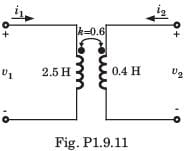Solution: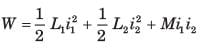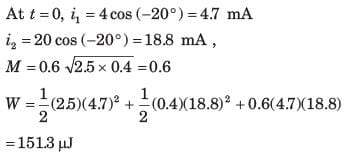QUESTION: 2

### Leq = ?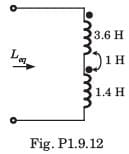Solution: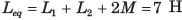QUESTION: 3

### Leq = ?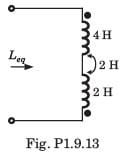Solution: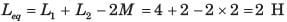QUESTION: 4

Leq = ?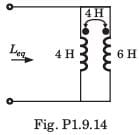Solution: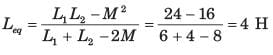QUESTION: 5

Leq = ?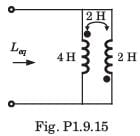Solution: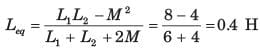QUESTION: 6

The equivalent inductance of a pair of a coupled inductor in various configuration are
(a) 7 H after series adding connection
(b) 1.8 H after series opposing connection
(c) 0.5 H after parallel connection with dotted terminal connected together.
The value of L1 ,L2 and M are

Solution: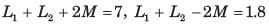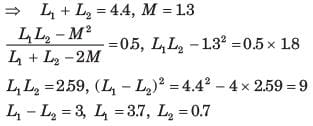QUESTION: 7

Leq = ?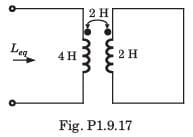Solution: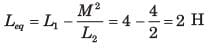QUESTION: 8

Leq = ?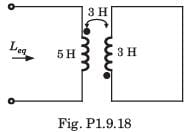Solution: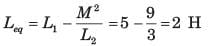QUESTION: 9

In the network of fig. P1.9.19 following terminal are connected together
(i) none (ii) A to B (iii) B to C (iv) A to C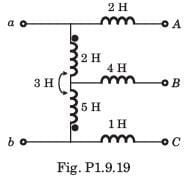The correct match for equivalent induction seen at terminal a - b is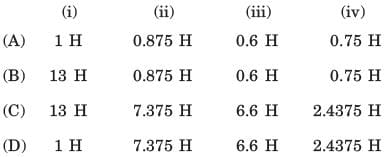Solution:QUESTION: 10

Leq =?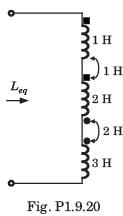Solution: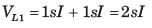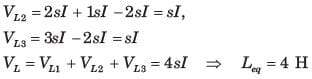Use Code STAYHOME200 and get INR 200 additional OFF Use Coupon Code